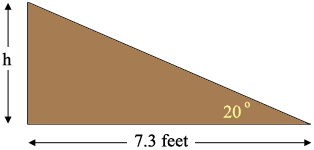SEARCH HOMEMath Central Quandaries & QueriesQuestion from christine, a parent: A roof on a shed is 7.3 ft wide has an incline of 20 degrees what is the height?Hi Christine,

Is this the diagram you have in mind where you want o find $h$?If so then what trig functions related the angle measurement, $20^o$ and the side lengths $h$ feet and 7.3 feet?

PennyMath Central is supported by the University of Regina and The Pacific Institute for the Mathematical Sciences.